# Thermodynamic Processes & Types

We know that if we have to take a thermodynamic system from the initial to the final state, we have several paths that can be taken. In this article, we will be discussing those thermodynamic processes. Before that, we will see what a quasi-static process is. It has been discussed that state variables are defined only when the thermodynamic system is in equilibrium with the surrounding. So a process in which at each moment the system is in thermodynamic equilibrium with the surrounding is known as a quasi-static process.

## The Thermodynamic Processes

### Isothermal Process:

It is a thermodynamic process in which temperature remains constant. We know,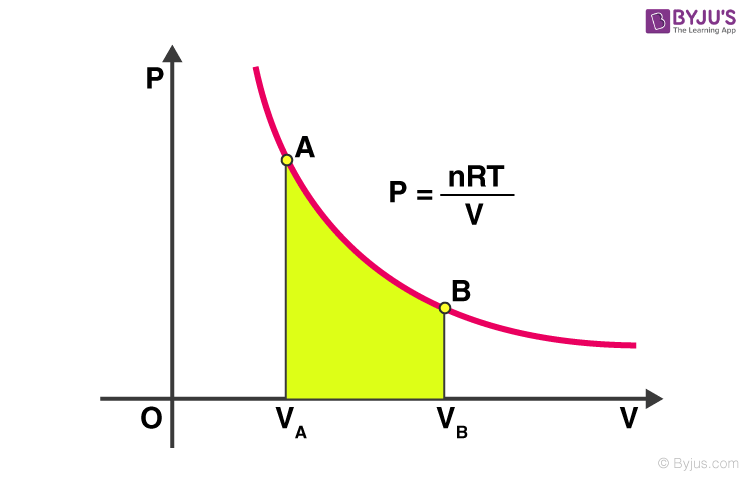$$\begin{array}{l}~~~~~~~~~~~~~~W = ∫P dV\end{array}$$

$$\begin{array}{l}~~~~~~~~~~~~~~According~~ to ~~Gas ~~law,\end{array}$$

$$\begin{array}{l}~~~~~~~~~~~~~~PV = nRT\end{array}$$

$$\begin{array}{l}~~~~~~~~~~~~~~\end{array}$$
$$\begin{array}{l} P = \frac{nRT}{v}\end{array}$$

Using this value of P in work done we get,

$$\begin{array}{l}~~~~~~~~~~~~~~\end{array}$$
$$\begin{array}{l} W = nRT∫_{V_A}^{V_B} \frac {dV}{V}\end{array}$$

$$\begin{array}{l}~~~~~~~~~~~~~~\end{array}$$
$$\begin{array}{l} W = nRT ln \frac{V_B}{V_A}\end{array}$$

If

$$\begin{array}{l} V_B > V_A\end{array}$$
work done is positive otherwise negative.
Also, we know internal energy only depends on temperature. As the temperature is constant hence ∆U = 0. So from first law of thermodynamics,

$$\begin{array}{l}~~~~~~~~~~~~~~Q = W\end{array}$$

It is a thermodynamic process in which no heat is exchanged between the system and the surrounding. So, Q = 0. Mathematically this process is represented as

$$\begin{array}{l}~~~~~~~~~~~~~~~~~~~~~~~~~~~~~~~~~\end{array}$$
$$\begin{array}{l}PV^γ=K(constant)\end{array}$$

$$\begin{array}{l}~~~~~~~~~~~~~~W = ∫P dV\end{array}$$

Substituting P we get,

$$\begin{array}{l}~~~~~~\end{array}$$

$$\begin{array}{l}~~~~~~~~~~~~~~\end{array}$$
$$\begin{array}{l} W = K \int^{V_f}_{V_i} \frac {dV}{V^γ} \end{array}$$

$$\begin{array}{l}~~~~~~~~~~~~~\end{array}$$
$$\begin{array}{l} W = K \frac{(V_f^{1-γ}- V_i^{1-γ})}{1-γ}\end{array}$$

$$\begin{array}{l}~~~~~~~~~~~~~~ ∆U = -W \end{array}$$

So if work done is negative internal energy increases and vice versa.

### Isochoric Process:

In isochoric process the change in volume of thermodynamic system is zero. As change in volume is zero so work done is zero. From First law,

$$\begin{array}{l}~~~~~~~~~~~~~~ Q = ∆U \end{array}$$

### Isobaric Process:

The pressure remains constant during this process. So ,

$$\begin{array}{l}~~~~~~~~~~~~~~\end{array}$$
$$\begin{array}{l} W = P (V_f- V_i)\end{array}$$

So if volume increases, work done is positive, else negative.

### Cyclic Process:

It is a process in which the final state of the system is equal to the initial state. As we know, change in internal energy is a state function, so, in this case, ∆U = 0.

## Thermodynamics Process Examples

1. Following is a PV curve showing two isothermal processes for two different temperatures. Identify the process that has a higher temperature.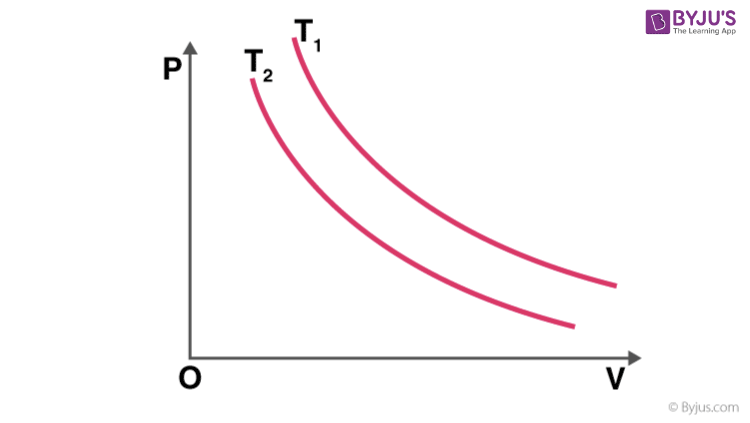Sol: To identify the process with higher temperature. First, a horizontal line must be drawn parallel to the x-axis. This horizontal line represents the constant pressure line.  Let Vand V2 be the volumes that belong to the same pressure as the vertical lines such that they meet the constant pressure line.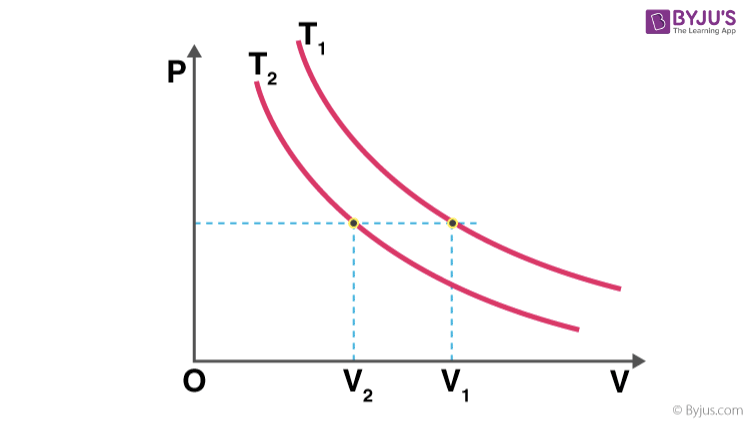We know that, at constant pressure, as the volume of the gas increases, the temperature also increases. From the above graph we can say that V> Vtherefore, T1 > T2. Also, an easier way to determine the temperature is that the curve close to the origin will have a lower temperature.

2. Following is a V-T graph for isobaric processes at two different pressures. Determine the curve that occurs at higher pressure.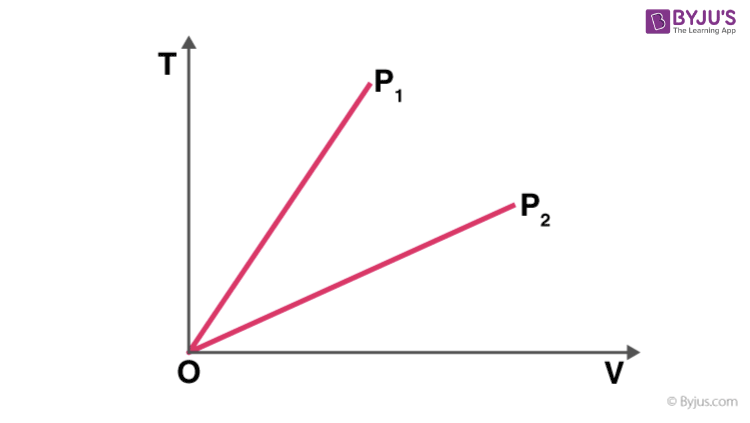Sol: From ideal gas equation, we get

$$\begin{array}{l}V=(\frac{nR}{P})T\end{array}$$

A volume-temperature graph is a straight line passing through the origin.

Also, the slope of the volume-temperature graph is

$$\begin{array}{l}(\frac{nR}{P})\end{array}$$

The slope of the graph is inversely proportional to the pressure. Therefore, if the slope is greater, the pressure will be lesser.

From the graph, it is clear that P1 has a larger slope than P2. Therefore, P2 > P1.

The video compares different thermodynamic processes graphically.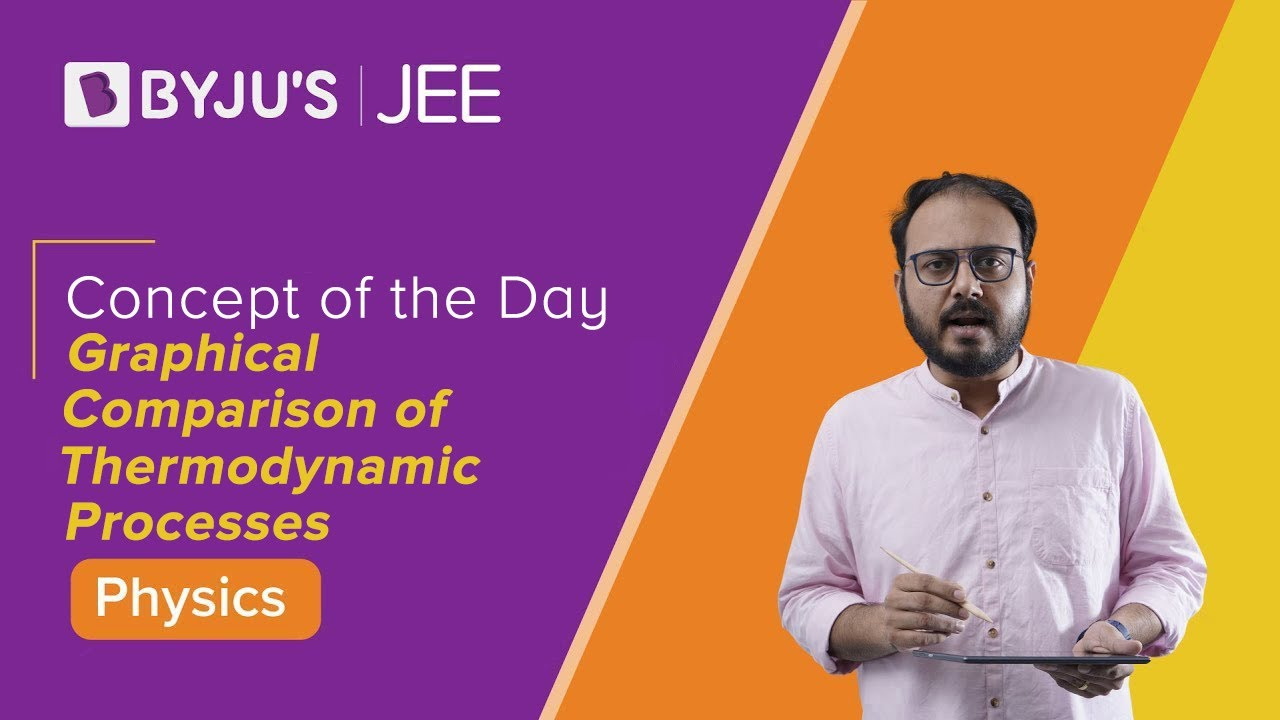### When do all gases and vapours approach ideal gas behaviour?

For all gases and vapours to approach ideal gas behaviour, they need low pressure and low density.

### What is the triple point of water in degree Celsius?

The triple point of water is defined as the temperature and pressure at which the solid, liquid, and gaseous states of water are in equilibrium with each other. 0.01 degree Celsius is the triple point of water.

### State if the given statement is true or false: The enthalpy of an ideal gas depends only on the temperature.

The given statement is true because the internal energy of an ideal gas depends only on the temperature.

### State if the given statement is true or false: Enthalpy is an intensive property of a system.

The given statement is true. An intensive property is defined as the property of matter which is independent of the amount of matter.

### What happens to the state of the substance at a pressure below the triple point?

When the substance is at a pressure below the triple point, it cannot exist in the liquid state, and when the substance is heated, it transforms from solid to vapour.

Test Your Knowledge On Various Processes In A Thermodynamic System!